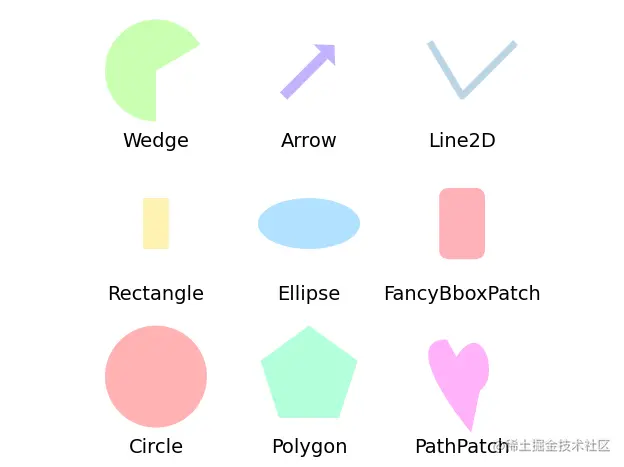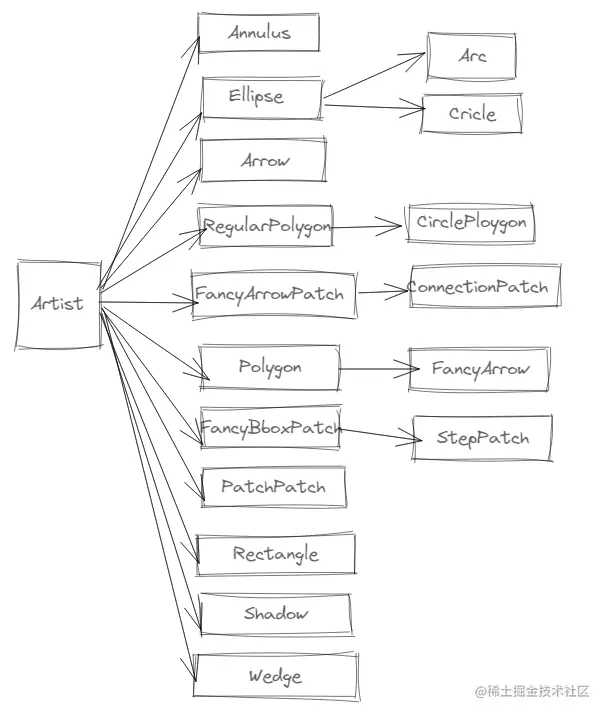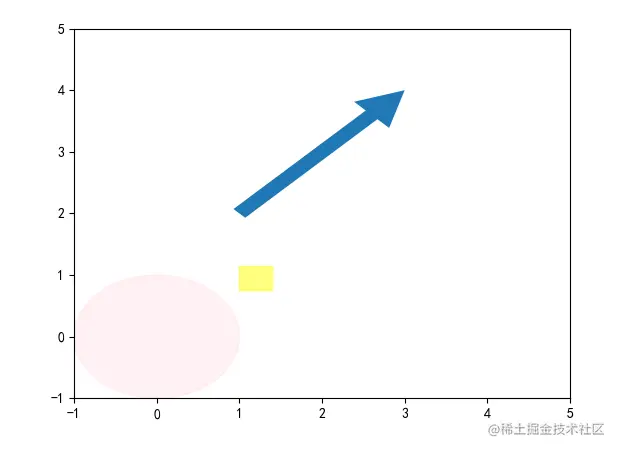# Python Matplotlib drawing graphics

2022-02-01 00:07:48This is my participation 11 The fourth of the yuegengwen challenge 18 God , Check out the activity details ：2021 One last more challenge

# Preface

We are facing matplotlib Module underlying structure learning , For its pyplot class （ Script layer ） Class to draw a line chart 、 Histogram 、 The pie chart 、 Histogram and other statistical charts , List previous articles as follows .

matplotlib The module not only provides the function of drawing statistical charts , It also supports drawing circles 、 Square 、 Rectangle and other graphicsIn this issue , Let's learn to use matplotlib Module draw common graphics ,Let's go~

# 1. matplotlib.patches summary

matplotlib.patches A class dedicated to drawing graphics , In this class, we use Artist Base class

• pathes It's specially drawn 2D Graphic class
• patch The drawing defaults to rc params Set up
• patch The module provides up to 10 A graphic method to meet daily needs# 2. Drawing method

Corresponding matplotlib The module says ,patches Class to draw a circle 、 ellipse 、 Rectangle and other graphic methods

Method effect
patches.Rectangle(xy,width,height,angle=0) Draw a rectangle
patches.Polygon(xy) Draw polygon
patches.Arc(xy,width,height,angle=0) Draw an ellipse
patches.Ellipse(xy,width,height,angle=0.0) Draw an ellipse
patches.wedge(center,r,theta1,theta2,width) Draw a wedge
patches.PathPatch() Draw a multi curve graph
patches.FancyBboxPatch() Draw a fancy graphic box
patches.Line2D() Draw lines

# 3. Drawing graphics steps

stay matplotlib Module , The charts are made of figure、Axes and Axis Three basic elements , So when drawing graphics , The general steps are mainly composed of the following .

• Import matplotlib pyplot and patches class
``````import matplotlib.pyplot as plt
import matplotlib.patches as mpatch
Copy code ``````
• Use subplots() Create subgraphs Axes object
``````fig,ax =plt.subplots()
Copy code ``````
• call pathes Class to draw graphics, such as drawing a rectangle Rectangle()
``````Rect = mpatch.Rectangle((0.2,0.75),0.4,0.4,color="r")
Copy code ``````
• Subgraphs Axes Object call set_xlim() and set_ylim Axis range

patches By default ,x The coordinate range of the axis is （0,1）,y The coordinate range of the axis is （0,1）

``````ax.set_xlim(-2,5)
ax.set_ylim(-2,5)
Copy code ``````
``````ax.add_patch(Rect)
Copy code ``````
• call pyplot.show() Show graphics

# 4. Draw graphic properties

• ## Set transparency

• keyword ：alpha
• The value type is ： floating-point
• ## Set the color

• Set graphic keywords ：color
• Set border keyword ：edgecolor
• The value is optional ：
• English words for color ： Like red "red"
• Abbreviations of words indicating color, such as ： Red "r", yellow "y"
• RGB Format ： Hexadecimal format, such as "#88c999";(r,g,b) Tuple form

# 5. A profound

After learning the above sections , We draw circles in the chart 、 Rectangles and straight lines

``````def drawpicture():

fig,ax =plt.subplots()

Rect = mpatch.Rectangle((1,0.75),0.4,0.4,color="yellow",alpha=0.5)

Cri = mpatch.Circle((0,0),1,angle=30,color="pink",alpha=0.2,capstyle="round")

Py = mpatch.Arrow(1,2,2,2)

ax.set_xlim(-1,5)
ax.set_ylim(-1,5)

plt.show()

drawpicture()
Copy code ``````# summary

In this issue , We are right. matplotlib Draw graphics related methods and steps to learn , In practice , It takes a lot of practice to use... More skillfully

The above is the content of this issue , Welcome big guys to praise and comment , See you next time ~